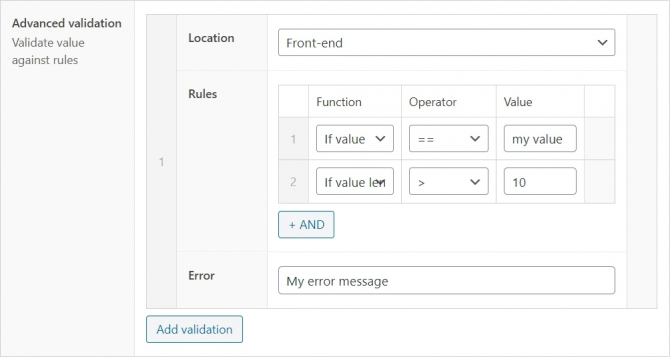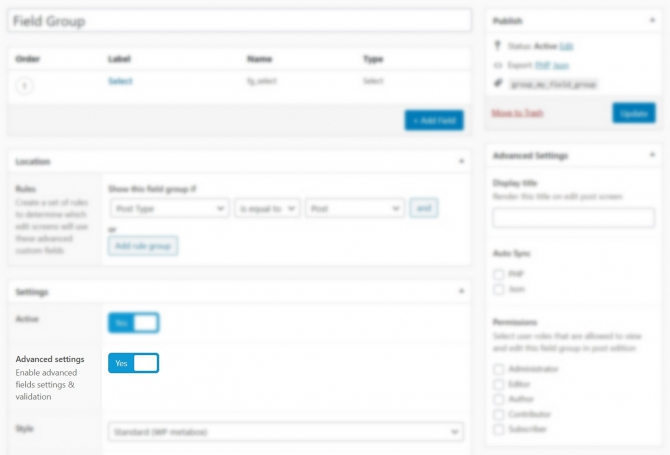﻿ Advanced Validation – ACF Extended

Define custom validation rules and error messages based on the current screen location in the field’s UI.### Getting Started

In order to enable the Advanced Validation UI, you must enable the “Advanced Settings” setting in the field group and save it. The Advanced Settings UI will be available in all fields within that field group.### Available Locations

Choose the location screen where the validation rule should be applied. You can set multiple rules groups based on different locations.

• Everywhere
• Front-end

### Available Functions

The UI comes with predefined functions that can be used out of the box.

 Function name PHP Function If value `if(\$value)` If value length `if(strlen(\$value))` If count value `if(count(\$value))` If email exists `if(email_exists(\$value))` If post type exists `if(post_type_exists(\$value))` If taxonomy exists `if(taxonomy_exists(\$value))` If term exists `if(term_exists(\$value))` If username exists `if(username_exists(\$value))` If is email `if(is_email(\$value))` If is user logged in `if(is_user_logged_in())` If is array `if(is_array(\$value))` If is string `if(is_string(\$value))` If is numeric `if(is_numeric(\$value))` If get post type `if(get_post_type(\$value))` If post id exists `if(get_post_by_id(\$value))` If post slug exists `if(get_post_by_slug(\$value))` If post title exists `if(get_post_by_title(\$value))` If sanitize email `if(sanitize_email(\$value))` If sanitize file name `if(sanitize_file_name(\$value))` If sanitize html class `if(sanitize_html_class(\$value))` If sanitize key `if(sanitize_key(\$value))` If sanitize meta `if(sanitize_meta(\$value))` If sanitize mime type `if(sanitize_mime_type(\$value))` If sanitize option `if(sanitize_option(\$value))` If sanitize text field `if(sanitize_text_field(\$value))` If sanitize title `if(sanitize_title(\$value))` If sanitize user `if(sanitize_user(\$value))` If get user by id `if(get_user_by('id', \$value))` If get user by slug `if(get_user_by('slug', \$value))` If get user by email `if(get_user_by('email', \$value))` If get user by login `if(get_user_by('login', \$value))`

### Available Operators

The UI comes with predefined operators that can be used out of the box.

 operator == != > >= < <= Contains Doesn’t contain Starts with Doesn’t start with Ends with Doesn’t end with Matches regex Doesn’t match regex == true != true == false != false == null != null == “” (empty) != “” (empty)

### Set Validation Rules in PHP

It is possible to add validation rules based on the screen location programmatically using the `acf/validate_value` hook combined with `acfe_form_is_admin()` and `acfe_form_is_front()` functions.

Note that this method doesn’t require to enable the “Advanced Settings” in the field group as it is directly executed in PHP. See ACF documentation. Usage example:

``````/*
*/
function my_acf_validate_my_field(\$valid, \$value, \$field, \$input){

if(strlen(\$value) > 10)
\$valid = 'The value should contain less than 10 characters';

}

// Front-end only
if(acfe_form_is_front()){

if(strlen(\$value) > 30)
\$valid = 'The value should contain less than 30 characters';

}

// return
return \$valid;

}``````